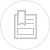# physics concepts of gases and thermodynamics

## ideal gases

An ideal gas satisfies the requirements of the Mariotte law (in an isothermal conversion, the product of the volume by the pressure is a constant) and of the Gay Lussac law (in a constant volume, the pressure of an ideal gas will be proportional to the absolute temperature).

### dalton law

This law governs the mixture of ideal gases: “pressure, internal energy, enthalpy and entropy of a combination of ideal gases will be respectively equal to the sum of the partial pressures, partial internal energies, partial enthalpies and partial entropies that the individual gases would have if each alone occupied the total volume at the temperature of the mixture”, i.e.:

This relation links the gramme-molecular weight M of a gas expressed in g·mol–1 to its specific gravity d in relation to air under normal pressure and temperature conditions.

### specific heat

By definition, the specific heat is the quotient of thermal capacity by mass, the thermal capacity being the quantity of heat dQ that has to be supplied to a system in order to increase the temperature by 1 °C.

For one gas, we have specific heat at constant pressure cp and specific heat at constant volume cv.

Find below the specific heat cp, at constant pressure for some gases in kJ/kg·°C at 0 °C and at 760 mm of mercury (table 85):

## water vapor

### saturating of saturated vapor

Vapor in the presence of the generating liquid phase; this vapor is termed dry when it does not contain a single droplet of water.

Vapor enthalpy: this is the total quantity of heat required to convert 1 kg of water taken at 0 °C into saturated steam at a temperature of t °C. It is the sum of the heat used to raise the temperature of the water from 0 to t °C (water enthalpy) and of the vaporisation heat at t °C equivalent to the necessary energy that has to be provided to convert 1 kg of water into 1 kg of vapour at t °C.

As an initial approximation and for temperatures of between 30 and 190 °C, the Regnault formula can be used, it provides the enthalpy according to the temperature expressed in °C:

• in kJ·kg–12 538 + 1.276 t;
• in kcal·kg–1606.5 + 0.305 t.

### wet vapor

Vapor containing droplets of water, characterised by its titer x: mass of vapor expressed in kg contained in 1 kg of the mixture.

### superheated vapor

Vapor having a temperature that is higher than that of the saturating vapor at the pressure concerned. As an initial approximation, it behaves like an ideal gas.

Superheated vapor enthalpy can be calculated using the following formula :

• in kJ·kg–1 2 538 + 1.276 t + cp (t – t1);
• in kcal·kg–1 606.5 + 0.305 t + cp (t – t1).

t – t1 being the difference in temperature between saturated vapor and superheated vapor at a constant pressure. As an initial approximation, we can use cp = 2.1 kJ·kg–1. In particular, this formula can be used to estimate the enthalpy of water that is evaporated in an incineration furnace from which the gases are discharged at a temperature t, in this case t1 being equal to 100 °C.

## wet gases

### definitions

#### dry temperature

Temperature of an unsaturated wet gas measured using a dry bulb thermometer (normal meaning of temperature).

#### wet temperature

Temperature of a wet gas that becomes saturated when in contact with a liquid film.

#### dew point

Temperature at which the vapor contained in the gas begins to condense through cooling at a given temperature.

#### saturating vapor pressure

Partial pressure of vapor in a gas at dew point.

#### relative humidity

Ratio between the partial pressure of the water vapor in the gas and the saturating vapor pressure equivalent to this gas’s dry temperature. It is usually expressed as a %.

### water vapor content of a gas (m)

also called "specific or absolute humidity". If P is the total pressure for a gas having a gramme-molecular weight M and partial vapour pressure pv, the water content m of a gas expressed in kg·kg–1 of dry gas, is provided by :

Thus, for air that is humidity saturated at 20 °C and at normal atmospheric pressure P = 1.013 bar; pv = 0.023 bar; m = 0.0147 kg·kg–1.

### wet gas enthalpy

Heat in mixtures can be regarded as negligible, the enthalpy for a wet gas will be equal to the sum of the enthalpies for the wet gas and of the vapour.

For air, the enthalpy is provided by the following formulae :